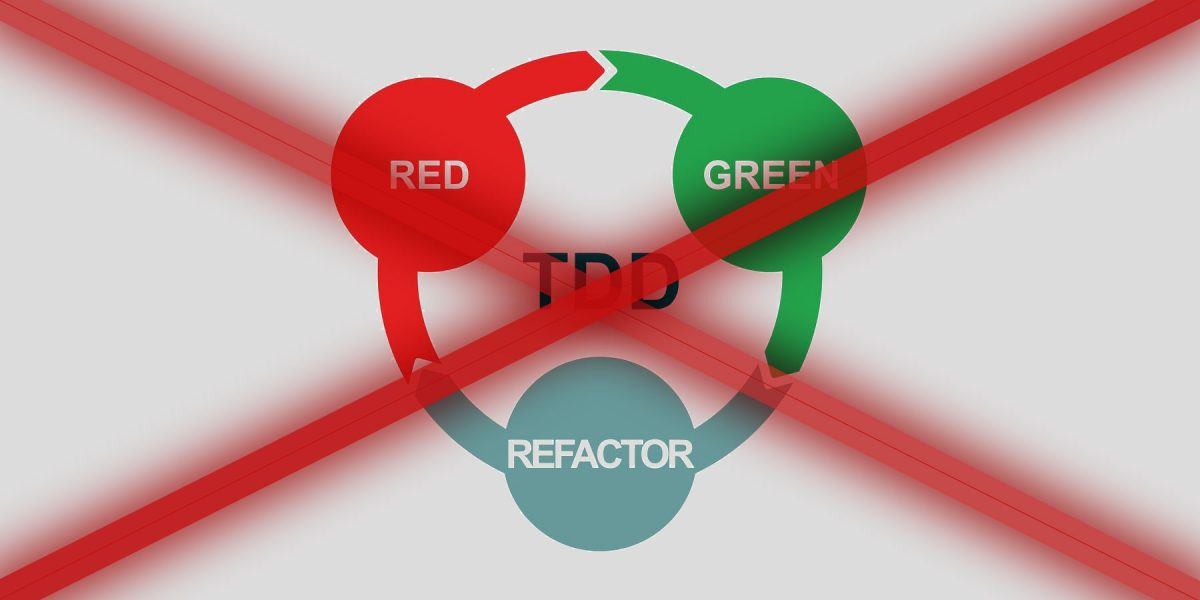# TDD: Test-driven development sucksDon’t click. The title is nothing more than clickbait.

When I’m starting writing this note is Saturday, and I’ve just come from a cafe. As usually, I went with my MacBook Pro and I’ve tried to create some code.

Today, I wanted to create a tool which helps me with mine investments on the stock market (Warsaw Stock Exchange).

## Stock

I know nothing about investments on the stock market. Honestly, I won’t know anything more than is necessary. I haven’t plan or ambition to be The Wolf of Wall Street. I want only to move a small part of my savings from a bank deposit to something more profitable. I assume I can lose this money, but as I said, I am investing only small amount.

I know nothing, I don’t want to learn and … I don’t want to lose time watching charts or exchange rates. How to invest with those requirements? Easy! Make some script which does everything that you don’t want.

## Behat scenario

On the beginning, I defined two rules when I should sell my stocks.

### Exchange rate is less than X\$

You buy stocks on some prices, and because you don’t want to lose too much, when a crisis comes, you need notification when rates will be too low.

```Feature: In order to notify when current value ETFSP500 dives
As a user
I want to declare lower limit
When notify will be sent.

Scenario: Current value and limit are integers.
When Current value is "65"
And Lower limit is "66"

Scenario: Current value and limit are fractions
When Current value is "65.08"
And Lower limit is "65.09"

Scenario: Current value is integer, and limit is fraction.
When Current value is "65"
And Lower limit is "65.01"

Scenario: Current value is fraction, and limit is integer.
When Current value is "65.99"
And Lower limit is "66"
```

### Exchange rate is less than average from last ten months

You are lucky guy, and stock market still grows. You are smart investor, and you know that you need exit strategy when crisis will come.

The easiest strategy is to resist on average stock exchange from last ten months. If the rate was lower than the average, you would sell what you collected.

```Feature: In order to notify when current value ETFSP500 is less than average from last ten months
As a user

# Integers
Scenario: Current value is equal average
and both numbers are integers.
When Current value is "65"
And Average is "65"

Scenario: Current value is lower than average
and both numbers are integers.
When Current value is "64"
And Average is "65"

Scenario: Current value is greater than average
and both numbers are integers.
When Current value is "66"
And Average is "65"

# Fractions
Scenario: Current value is equal average
and both numbers are fractions.
When Current value is "65.01"
And Average is "65.01"

Scenario: Current value is lower than average
and both numbers are fractions.
When Current value is "65.01"
And Average is "65.02"

Scenario: Current value is greater than average
and both numbers are fractions.
When Current value is "65.02"
And Average is "65.01"

# Current value is integer, and average is fraction.
Scenario: Current value is lower than average
and current value is integer, and average is fraction
When Current value is "65"
And Average is "65.01"

Scenario: Current value is greater than average
and current value is integer, and average is fraction
When Current value is "66"
And Average is "65.99"

# Current value is fraction, and average is fraction
Scenario: Current value is lower than average
and current value is fraction, and average is integer
When Current value is "65.99"
And Average is "66"

Scenario: Current value is greater than average
and current value is fraction, and average is integer
When Current value is "65.01"
And Average is "65"
```

## But why TDD sucks?

I went to cafe with small goal which was created one `spec` for `NotifierInterface`. Finally, I spent there a few hours. Why?

Do you know the flow in TDD?

1. Write test
2. Run test
3. Write implementation to test pass.

When you finish your work, you should leave one test without (or with broken) implementation. Therefore, you will know where you should start next time.

I couldn’t do that. Always, when I saw a red mark, that test was broken. I couldn’t leave it. I had to fix it and spent 3 minutes more, because and I can fix and verify it so fast!

Here, you can check what I’ve created in this longer than should be code session.## Author: Marcin Pilsniak

I have been using PHP for 13 years. Furthermore, for the last 4 years I have been using AWS in my everyday work. I’m certified AWS Architect, Developer and SysOps.

Posted on Categories UncategorizedTags